# Vue 进阶（幺叁幺）：父子组件传值实现数据深拷贝No Silver Bullet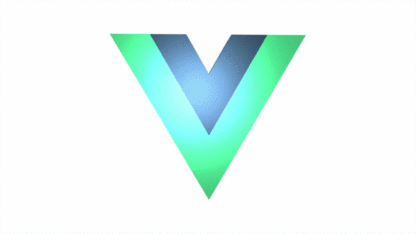## 二、问题分析

var a = [1,2,3];  var b = a; b.push(4); // b中添加了一个4alert(a); // a变成了[1,2,3,4]

 var obj = {a:10};  var obj2 = obj;  obj2.a = 20; // obj2.a改变了 alert(obj.a); // 20，obj的a跟着改变

computed: {          data: function () {                 var obj={};                  obj=JSON.parse(JSON.stringify(this.templateData)); //this.templateData是父组件传递的对象         return obj         }}

## 三、学海无涯

### 3.1 基本类型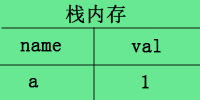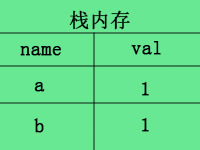### 3.2 引用数据类型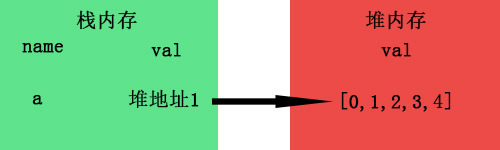b=a进行拷贝时，其实复制的是 a 的引用地址，而并非堆里面的值。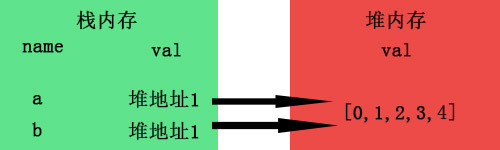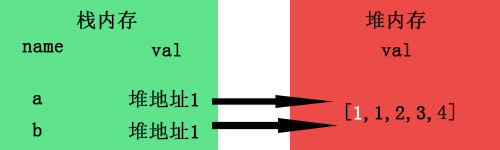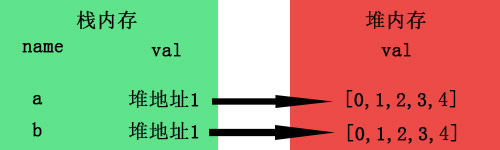## 四、深拷贝

### 4.1 递归

function deepClone(obj){    let objClone = Array.isArray(obj)?[]:{};    if(obj && typeof obj==="object"){        for(key in obj){            if(obj.hasOwnProperty(key)){                //判断ojb子元素是否为对象，如果是，递归复制                if(obj[key]&&typeof obj[key] ==="object"){                    objClone[key] = deepClone(obj[key]);                }else{                    //如果不是，简单复制                    objClone[key] = obj[key];                }            }        }    }    return objClone;}    let a=[1,2,3,4],    b=deepClone(a);a=2;console.log(a,b);

let a=[1,2,3,4],    b=a.slice();a=2;console.log(a,b);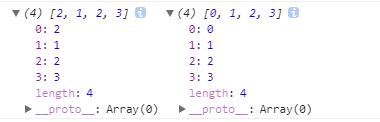let a=[0,1,[2,3],4],    b=a.slice();a=1;a=1;console.log(a,b);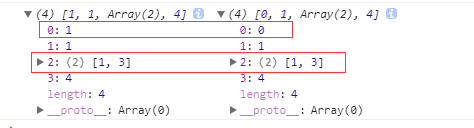### 4.2 parse 和 stringify

function deepClone(obj){    return JSON.parse(JSON.stringify(obj))}    let a=[0,1,[2,3],4],    b=deepClone(a);a=1;a=1;console.log(a,b);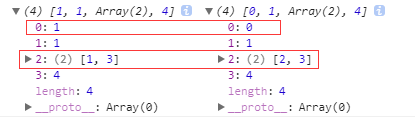### 4.3 extend

$.extend( [deep ], target, object1 [, objectN ] ) 复制代码 • deep表示是否深拷贝，为true为深拷贝，为false，则为浅拷贝。 • target Object 类型，目标对象，其他对象的成员属性将被附加到该对象上。 • object1 objectN 可选。 Object 类型，第一个以及第 N 个被合并的对象。 let a=[0,1,[2,3],4], b=$.extend(true,[],a);a=1;a=1;console.log(a,b);## 五、拓展阅读### No Silver Bullet

No Silver Bullet 2021.07.09 加入

## 评论